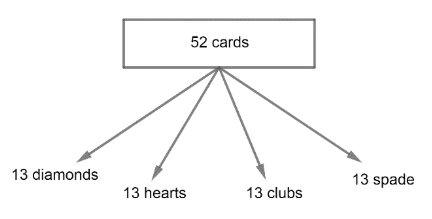Courses
Courses for Kids
Free study material
Free LIVE classes
MoreLIVE
Join Vedantu’s FREE Mastercalss

# 4 cards are drawn from a well-shuffled deck of 52 cards. What is the probability of obtaining 3 diamonds and one spade?Verified
360k+ views
Hint: We will first find the probability of selecting 4 cards out of 52 then we will find the probability of selecting 3 diamond cards out of 13 cards and then we will find the probability of selecting 1 spade card out of 13 cards and finally we will find the probability of selecting 3 diamond and 1 spade out of 52 cards.It is given in the question that 4 cards are drawn from a well-shuffled deck of 52 cards.
Also, it is given that out of those four selected cards 3 are diamond and 1 is a spade, and then we have to find the probability of obtaining 3 diamond cards and 1 spade from a well-shuffled deck of 52 cards.

So, the total number of cards is 52 and we have to select 4 cards out of 52.
We know that probability $=\dfrac{\text{Number of favourable outcomes}}{\text{Total number of outcomes}}$
So, total number of ways to select 4 cards out of 52 cards is $={}^{52}{{C}_{4}}$

We know that ${}^{n}{{C}_{r}}=\dfrac{n!}{r!\left( n-r \right)!}$ .
So, from this formula of ${}^{n}{{C}_{r}}$, we get,
\begin{align} & {}^{52}{{C}_{4}}=\dfrac{52!}{4!\left( 52-4 \right)!} \\ & =\dfrac{52!}{4!\left( 48 \right)!} \\ & =\dfrac{52\times 51\times 50\times 49\times 48!}{4!\times 48!} \\ \end{align}

Canceling the like terms we get,
\begin{align} & =\dfrac{52\times 51\times 50\times 49}{4!} \\ & =\dfrac{52\times 51\times 50\times 49}{4\times 3\times 2\times 1} \\ & =\dfrac{6497400}{24} \\ & =270725 \\ \end{align}

Also, we know that the total number of diamond cards is 13.
And we have to select 3 cards out of 13 cards.

So, the probability of selecting 3 diamond cards out of 13 diamond cards is $={}^{13}{{C}_{3}}$.
Similarly, we have a total of 13 spade cards and we have to select 1 card out of 13 cards.
So, the probability of selecting 1 spade card out of 13 spade cards is $={}^{13}{{C}_{1}}$.

Now, we have all the required data to find the probability of obtaining 3 diamond cards and 1 spade card from a well-shuffled deck of 52 cards.
So, the probability of obtaining 3 diamond cards and 1 spade card,
$=\dfrac{\text{Number of ways to select 3 diamond}\times \text{ Number of ways to select 1 spade}}{\text{Total ways to select 4 cards out of 52 cards}}$

Now, putting the value of the number of ways to select 3 diamond as ${}^{13}{{C}_{3}}$, number of ways to select 1 spade as ${}^{13}{{C}_{1}}$ and total ways to select 4 cards out of 52 cards as ${}^{52}{{C}_{4}}$we get,
Probability of obtaining 3 diamond cards and 1 spade card $=\dfrac{{}^{13}{{C}_{3}}\times {}^{13}{{C}_{1}}}{{}^{52}{{C}_{4}}}$.
Using formula ${}^{n}{{C}_{r}}=\dfrac{n!}{r!\left( n-r \right)!}$, we get,
\begin{align} & =\dfrac{\dfrac{13!}{3!\times \left( 13-3 \right)!}\times \dfrac{13!}{1!\times \left( 13-1 \right)!}}{\dfrac{52!}{4!\times \left( 52-4 \right)!}} \\ & =\dfrac{\dfrac{13!}{3!\times 10!}\times \dfrac{13!}{1!\times 12!}}{\dfrac{52!}{4!\times 48!}} \\ & =\dfrac{\dfrac{13\times 12\times 11\times 10!}{3!\times 10!}\times \dfrac{13\times 12!}{1!\times 12!}}{\dfrac{52\times 51\times 50\times 49\times 48!}{4!\times 48!}} \\ \end{align}

Canceling the like terms we get,
\begin{align} & =\dfrac{13\times 12\times 11}{3!}\times \dfrac{13}{1}\times \dfrac{4\times 3!}{52\times 51\times 50\times 49} \\ & =\dfrac{13\times 12\times 11\times 13\times 4}{52\times 51\times 50\times 49} \\ & =\dfrac{89232}{6497400} \\ \end{align}

Dividing numerator and denominator by 312, we get,
\begin{align} & =\dfrac{\dfrac{89232}{312}}{\dfrac{6497400}{312}} \\ & =\dfrac{286}{20825} \\ \end{align}

Thus, the probability of selecting 3 diamond cards and one spade card out of a well-shuffled deck of 52 cards is $\dfrac{286}{20825}$.

Note: In this type of question do not calculate any basic calculation individually but try to solve this by bringing them together in operation. This is because many times we get similar terms in the final calculation and they get to cancel out and because of this our calculation becomes easy and short, this will save our time.
Last updated date: 20th Sep 2023
Total views: 360k
Views today: 9.60k